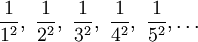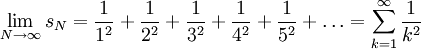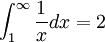# A Motivating Problem for The Integral Test

Previous: The Integral Test

Next: A Second Motivating Problem for The Integral Test

## Example

Does the following seriesconverge? The method of determining whether this series converges only requires a few steps, but first let's explore this problem using the tools we already have to see why the integral test is needed.

In practice, determining whether an infinite series converges is not a matter of simply applying an algorithm that can be memorized. Rather, we must try different approaches that we know and sometimes develop a new approach to solve a problem.

## Can We Use the Divergence Test?

Realistically, when students are working on infinite series problems on their own, they often encounter situations where the test they want to apply does not provide the information they are looking for. For example, applying the divergence test on this series, we find that the limitequals zero. So the divergence test yields no information, and we must use another test.

In this lesson we will introduce a method that can be used to establish that it does using the Integral Test.

## Relating Our Series to an Integral

As you might have guessed, the integral test makes a connection between an infinite series and an integral. To introduce an integral, we must introduce a function to integrate. What function do you think we could use?

Because the general term of our series is 1/k2, let's try the function f(x) = 1/x2. It will be helpful to note here that this function is greater than zero for all x.

Let's also recognize that an integral of a function, that is non-negative, can be interpreted as an area, so we can look for a geometrical (or graphical) interpretation of the given problem that involves areas.The function 1/x and rectangles approximating the area under the curve. In this example, the rectangles touch the curve at the upper right corner.

In the figure we have a graph of f(x) = 1/x2 along with a set of rectangles. Notice that the areas of the rectangles areBy construction, the width of each rectangle is 1. Adding the first N areas together we obtain the partial sum,and taking the limit as N goes to infinity we obtain an infinite series,This infinite series is exactly the infinite series that we were given. Also observe that

1. because the areas are all positive, the partial sum is an increasing sequence, and
2. the infinite sequence, equal to sum of the areas of the rectangles, is less than the total area under the curve

Indeed, the total area under the curve is given by the integralFinally, because we have an increasing sequence, sn that has a finite upper bound, we know that the given sequence must be convergent.

Our final result uses the Monotonic Sequence Theorem for sequences which the reader may not have encountered, but is straightforward. The diagram below demonstrates the concept of the theorem, which is also described in an appendix.

## What Assumptions Did We Need?

To solve our problem, we needed to make two assumptions. These assumptions are given later in this lesson, but try to go back through our example to uncover what special requirements were needed to get to our result. These assumptions lead us to the necessary conditions to apply the integral test.

Previous: The Integral Test

Next: A Second Motivating Problem for The Integral Test